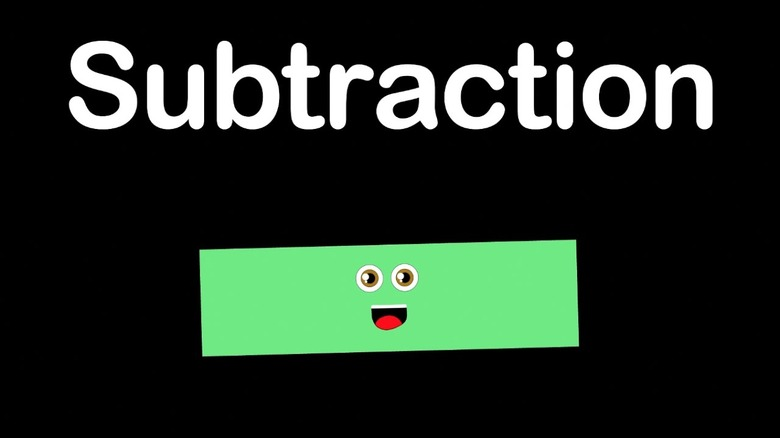Author:
Cindy Jordan
Subject:
Elementary Mathematics
Material Type:
Lesson
Level:
Lower Elementary
Tags:
Base Ten Blocks, Lesson Plan, Regrouping, Subtraction
Creative Commons Attribution Non-Commercial Share Alike
Language:
English
Media Formats:
Text/HTML, Video

# Subtraction with regrouping## Overview

This lesson plan is designed to provide a resourch for teachers who have students needing extra support with subtraction and regrouping through the place value chart up to 1,000. It primarily targets using base ten blocks to help students visualize and manipulate regrouping.

# Summary: regrouping with subtraction

This is a supplement lesson for reteaching subtraction with regrouping for students not meeting benchmarks. Teachers use a pre-test to identify struggling students. Can also be used for preteaching purpose.

Five day lesson with small group or one on one for 5 - 10 minutes long.

Using formative and pre- assessment data to determine students and needs provided by teacher's anthology

Formative assessment consist of check progresse worksheets provided by anthology and teacher observance in 5 day target plan.  Day 1-4 consist of modeling with base ten blocks, draw a model of base ten blocks, using algorithm, and checking with addition. Day 5 written formative assessment and finally mastery connect assessment (online)

# Background for Teachers

In this lesson, you will need an understanding of what concept the student is confused on. Using the formative and pre-test, pinpoint the errors commonly missed by the student. You will need a clear understanding of demonstrating subtraction using base ten blocks. ( youtube video attached below). Use of common vocabulary such as: difference, take away, subtract, regroup, more, less, expanded form, vertical, and place value. Materials needed: whiteboard with marker, base ten blocks, emedia video, paper, pencil, place value chart (optional), and worksheet with math problems below.

Reteaching subtraction

1.56-15=                         2. 47-28=                3. 73-24=

4. 65-39=                        5. 143-25=                6. 184-67=

7. 192-64=                        8. 227-38=                9. 385-99=

10. 216=38=                11. 142-125=        12. 381-292=

13. 1,344-196=                14. 1,460-559=

15. 1,210-847=                 16. 2,170-468=

Place value chart:

 thousands hundreds tens ones

# Step 1 - Goals and OutcomesLearning Intentions:

• Students will be able to Fluently subtract within 1,000 using strategies and algorithms based on place value, properties of operations, and/or the relationship between addition and subtraction

• Success Criteria:
• Students will correctly answer 80% of given problems. They will demonstrate using addition that problem is correct. Students will use correct vocabulary: difference, more, less, equal, take away, vertical

# Step 2 - Planning InstructionStudent Background Knowledge

• Prior to this lesson, students will need to have an understanding of the place value chart, do regrouping with addition up to the 1,000 and fluently subtract without regrouping.

Strategies for Diverse Learners

This is a lesson designed for students struggling with subtraction with regrouing. Once teacher has determined student need, they can use base ten blocks to model subtraction with regrouping. Some students will start with regrouping the tens, then move to hundreds, then thousands, and finally with zeros. Students start with base ten blocks and move to drawing the models then using the alogrithm.  Students will use addition to check for correctness

# Step 3 - Instruction

Using data from formative and pre-test assessments, select students who need additional help with subtracting with regrouping. After student selection, bring small group or one on one to work with you. Provide a whiteboard, marker and base ten blocks. Each day provide students a question:  write question vertically,  use base ten blocks, model drawing base ten blocks, use algorithm. check with addition. Have students talk aloud using vocabulary demonstrating their thinking.

Day 1 Begin by showing the emedia video starting with regrouping by tens, pause and provide students with questions to solve on their own, (example question provided in teacher background).

Day 2 continue with emedia video to hundreds place as above (pause). Have students check 1 question using addition. See if student can read the math problem correctly. ex. 45 from 126 or 126 minus 45. Provide students opportunity to talk aloud their thinking.

Day 3 continue with emedia video subtracting to thousands place (pause). Today have student use base ten blocks for first question then have them draw out a model of using base ten blocks for 2 questions.  Have student talk out the thinking.  Help with using correct vocabulary: difference, more, less, take away, equal, ect.  Check one with addition.

Day 4 finish emedia video working with zeros. Review past concepts, subtract with regrouping tens, hundreds, thousands. start with base ten blocks, draw a model of base ten blocks, use algorithm. check with addition

Day 5 assessment of 10 questions. Students correctly answer 80%

Mastery connect test 80% correct

# Step 4 - Assessments

During each day of one on one or group work, provide student opportunities to talk through their thinking. This will provide you informaton on what they know and misunderstandings.

End the week with this formative assessment to check for understanding on their own. Have students select 3 questions and check with addition. If student receives 80%, give the mastery connect test.

Name _______________

Reteaching subtraction assessment.

Write each question out vertically and show your work. Check three using addition.

1.76-35=                         2. 67-48=                3. 143-34=

4. 265-139=                        5. 183-85=                6. 1,284-169=

7. 1,192-164=                8. 1,240-159=

9. 1,380-909=                10. 2,210=438=

Place value chart: Fill in the words: tens, thousands, ones, hundreds

 ,